# Db2 Analytics Accelerator for z/OS## DB2## Data and AI on IBM Z

View Only

### How to calculate uncompressed Db2 for z/OS table sizes

#### By Pat Zakhar posted Wed April 29, 2020 02:17 PM

Written by Mehmet Cuneyt Goksu, PhD

Calculating the uncompressed table size for Db2 for z/OS is sometimes required for Db2 Analytics Accelerator sizing, capacity planning of Db2 for z/OS infrastructure and planning the future growth of the IBM Z platform. This blog post provides a step by step instructions to calculate the uncompressed table size.
How to calculate uncompressed Db2 for z/OS table sizes

This document provides step-by-step instructions on how to calculate uncompressed table sizes in a Db2 for z/OS environment. This calculation is required for Db2 Analytics Accelerator sizing, capacity planning of the Db2 for z/OS infrastructure and planning of the IBM Z platform growth.

## Db2 for z/OS compression overview

Db2 for z/OS provides the option to compress a table during table creation or later. Compression is enabled with the COMPRESS YES parameter on the tablespace level, during CREATE, or ALTER TABLESPACE DDL.

CREATE TABLESPACE tablespace_name … COMPRESS YES …

ALTER TABLESPACE tablespace_name … COMPRESS YES …

 Catalog Table Column Description SYSTABLEPART COMPRESS “Y”: compression used, “blank”: compression not used; can be at partition level SYSTABLEPART PAGESAVE % of pages saved 0 No savings SYSTABLEPART AVGROWLEN Average row length with or without compression SYSTABLES PCTROWCOMP % of compressed rows within total number of active rows

In general, it is recommended that you schedule REORG, RUNSTATS and STOSPACE runs  to keep compression statistics on the tablespaces and in the Db2 for z/OS catalog up-to- date.

There is a common misunderstanding regarding the calculation. For instance, the SPACE column in the SYSIBM.SYSTABLEPART table shows the number of kilobytes of DASD storage allocated to the tablespace partition, as determined by the last execution of the STOSPACE utility or RUNSTATS utility. However, this may be misleading for a calculation of the uncompressed table size. Firstly, this is the allocated space and not the real data size in the tablespace; secondly, if it is a compressed tablespace, this is the value of the compressed data.

## Calculation setup

The following example provides step-by-step information on how to calculate the uncompressed data:

Figure 1: Simple database / tablespace / table setupFigure 2: CUTB is loaded with 4607 records, and the VSAM cluster looks as follows: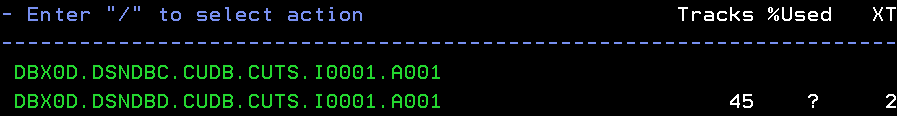Figure 3: The CUDB.CUTS tablespace uses 45 tracks on the DASD, and this is the value you find in the Db2 catalog. 45 Tracks is approximately 2,160 KB. The PAGESAVE ratio is %82.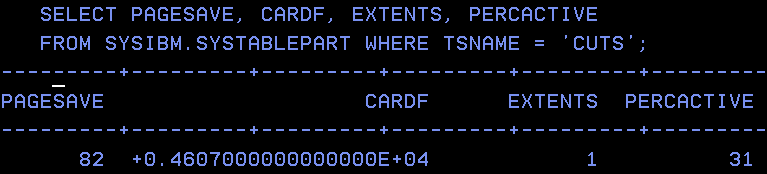## Calculation steps

Step 1: The real ‘Used space’ value, in bytes, is stored in the HI-U-RBA column of the VSAM cluster, which is a highly used relative byte address – the actual space occupied by the VSAM dataset.

The HI-U-RBA value is made visible through the LISTCAT TSO command:

<LISTC ENT(/) ALL>

It is used as shown below: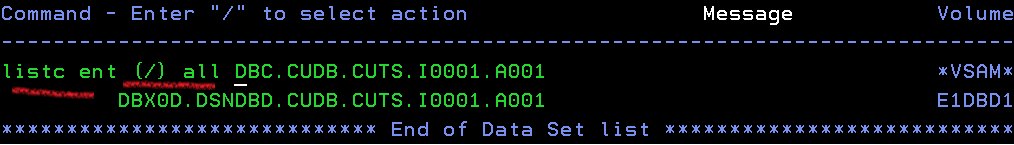In the command output, you view the HI-U-RBA value: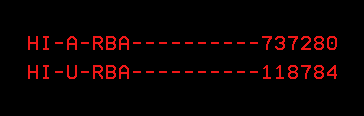Step 2: Find the PAGESAVE value in the SYSIBM.SYTABLEPART table for the tablespace. As shown in figure 3 above, this value can easily be found with a Db2 catalog query.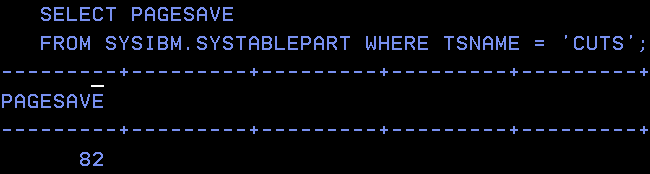Step 3: The formula for the actual uncompressed size of the tablespace is:

(HI-U-RBA / (100 - PAGESAVE)) * 100

In the above example, the HI-U-RBA value is 118,784
bytes, which is the compressed and the used space for the tablespace.

Then the values are applied to the formula as follows:

(118784 / (100 – 82)) x 100 = 659,911 bytes, which is the uncompressed size of the table.

Therefore, the size of compressed table is 118,784 bytes, and the uncompressed size of that object is 659,911 bytes.

Step 4:

• Apply the formula in step 3 to the TABLESPACEs defined with COMPRESS YES, then calculate the total uncompressed table size for compressed tables.
• Calculate the sum of all HI-U-RBA values of all tablespaces defined with COMPRESS NO, which gives you the total uncompressed table size for uncompressed tables.
• The total of both calculations above gives you the total uncompressed table size in Db2 for z/OS.